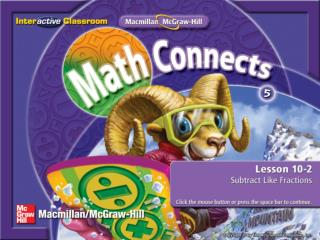DownloadDownload PresentationSplash Screen

# Splash Screen

Download Presentation## Splash Screen

- - - - - - - - - - - - - - - - - - - - - - - - - - - E N D - - - - - - - - - - - - - - - - - - - - - - - - - - -
##### Presentation Transcript

1. Splash Screen

2. Five-Minute Check (over Lesson 10–1) Main Idea Example 1: Subtract Like Fractions Key Concept: Subtract Like Fractions Example 2: Subtract Like Fractions Example 3: Subtract Like Fractions Lesson Menu

3. I will subtract fractions with like denominators. Main Idea/Vocabulary

4. Find – . Use models to check. 9 6 10 10 9 6 10 10 3 3 10 10 9 6 3 Answer: So, – = . 10 10 10 Subtract Like Fractions 9 – 6 – = 10 = Subtract. Example 1

5. A B C D 7 3 Find – . 11 11 1 A. 11 3 B. 10 4 C. 11 3 D. 0 Example 1

6. KC 1

7. Robert used teaspoon of salt and teaspoon of pepper in a recipe. How much more salt did he use than pepper? Write in simplest form. 3 1 4 4 Subtract Like Fractions Subtract the amount of pepper in the recipe from the amount of salt. Example 2

8. 3 1 1 2 4 2 4 4 1 Answer: So, Robert used teaspoon more salt than pepper. 2 Subtract Like Fractions 3 – 1 Subtract the numerators. = – 4 Simplify. = Divide the numerator and denominator by the GCF, 2. 2 ÷ 2 = 4 ÷ 2 Simplify. Check by drawing a picture. = Example 2

9. A B C D Melanie used cup of milk and cup of flour in a recipe. How much more milk did she use than flour? 4 1 6 6 1 A. cup 3 1 B. cup 2 2 C. cup 3 1 D. cup 6 Example 2

10. Nadia used foot of string for a necklace and Kate used foot of string. How many fewer feet of string did Kate use than Nadia? Write in simplest form. 11 12 8 12 Subtract Like Fractions Subtract the amount of string that Kate used from the amount of string that Nadia used. Example 3

11. 11 8 1 12 12 4 3 12 1 Answer: So, Kate used foot less string than Nadia. 4 Subtract Like Fractions 11 – 8 Subtract the numerators. = – 12 Simplify. = Divide the numerator and denominator by the GCF, 3. 3 ÷ 3 = 12 ÷ 3 Simplify. Check by drawing a picture. = Example 3

12. A B C D Find – . Which is the answer in simplest form? 7 1 10 10 6 A. 10 3 B. 5 1 C. 4 2 D. 3 Example 3

13. End of the Lesson End Lesson

14. Five-Minute Check (over Lesson 10–1) Image Bank Math Tool Chest Add Like Fractions Resources

15. A B C D 3 A. 10 5 B. 15 4 C. 5 3 D. 5 (over Lesson 10–1) Five Minute Check 1

16. A B C D 3 C. 14 5 B. 14 5 A. 7 2 D. 7 (over Lesson 10–1) Five Minute Check 2

17. A B C D 6 D. 12 4 A. 12 1 B. 3 2 C. 3 (over Lesson 10–1) Five Minute Check 3

18. A B C D 4 A. 12 1 2 B. D. 3 3 3 C. 4 (over Lesson 10–1) Five Minute Check 4

19. A B C D 9 A. 18 1 B. 2 2 D. 3 (over Lesson 10–1) C.1 Five Minute Check 5

20. A B C D 2 A.1 3 6 1 B. D.1 8 2 3 C. 4 (over Lesson 10–1) Five Minute Check 6

21. End of Custom Shows